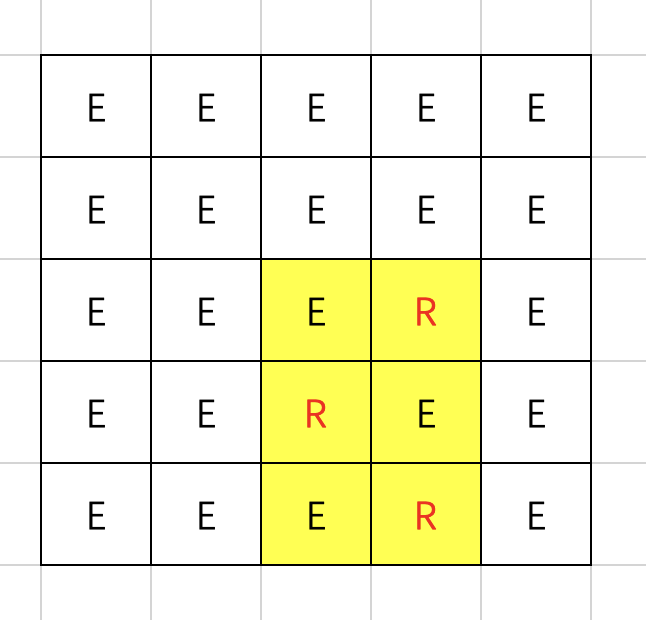# A. Minimums and Maximums

## 题目大意

• 在这个数组中至少有$l_1$且至多有$r_1$个元素的值等于数组中的最小值。
• 在这个数组中至少有$l_2$且至多有$r_2$个元素的值等于数组中的最大值。

# B. Robots

## 题目解析# C. Binary String

## 题目大意

• 操作完成之后，字符串中剩余0的数目。
• 操作完成之后，字符串中移除1的数目。

# D. Dog Walking

## 题目大意

• $a_i < 0$ 代表🐶往左走了 $|a_i|$ 米。
• $a_i = 0$ 不确定🐶怎么走。(Unknown)
• $a_i > 0$ 代表🐶往右走了 $|a_i|$ 米。

$n$ 分钟之后，🐶会回到你身边，就是位置为 $0$ 的地方。

# E. Moving Chips

## 题目解析

• dpl[i][j - 1] 将包含 $j - 1$ 列的所有左边的棋子转移到当前位置。
dpl[i][j] = dp[i][j - 1] + 1
如果 $i \oplus 1, j$ 位置存在一枚棋子，那么也需要转移过来。dpl[i][j]++
• dpl[i^1][j - 1] 将包含 $j - 1$ 列的所有左边的棋子转移到当前位置。
dpl[i][j] = dp[i^1][j - 1] + 2
如果 $j - 1$ 列及左边没有棋子，这种情况包含在上面一种情况当中了，就不再考虑。

## 参考代码

PS: 代码中的 dpl[i][j], dpr[i][j]的两维和上述分析中不同。

Educational Codeforces Round 128 (Rated for Div. 2)
https://dianhsu.com/2022/05/14/cf1680/

Dian Hsu

2022年5月14日